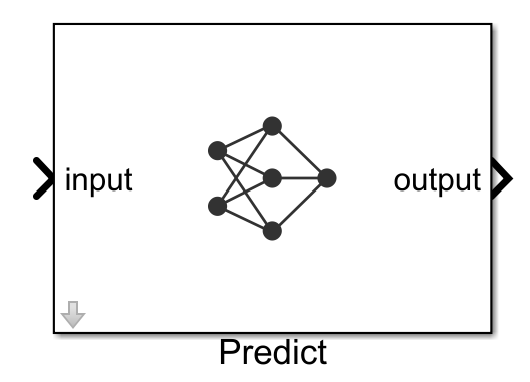# Predict

Predict responses using a trained deep learning neural network

• Library:
• Deep Learning Toolbox / Deep Neural Networks

•## Description

The Predict block predicts responses for the data at the input by using the trained network specified through the block parameter. This block allows loading of a pretrained network into the Simulink® model from a MAT-file or from a MATLAB® function.

Note

Use the Predict block to make predictions in Simulink. To make predictions programmatically using MATLAB code, use the `classify` and `predict` functions.

## Ports

### Input

expand all

The input ports of the Predict block takes the names of the input layers of the network loaded. For example, if you specify `googlenet` for `MATLAB function`, then the input port of the Predict block is labeled data. Based on the network loaded, the input to the predict block can be image, sequence, or time series data.

The format of the input depend on the type of data.

DataFormat of Predictors
2-D imagesA h-by-w-by-c-by-N numeric array, where h, w, and c are the height, width, and number of channels of the images, respectively, and N is the number of images.
Vector sequencec-by-s matrices, where c is the number of features of the sequences and s is the sequence length.
2-D image sequencesh-by-w-by-c-by-s arrays, where h, w, and c correspond to the height, width, and number of channels of the images, respectively, and s is the sequence length.
FeaturesA N-by-`numFeatures` numeric array, where N is the number of observations and `numFeatures` is the number of features of the input data.

If the array contains `NaN`s, then they are propagated through the network.

### Output

expand all

The outputs port of the Predict block takes the names of the output layers of the network loaded. For example, if you specify `googlenet` for `MATLAB function`, then the output port of the Predict block is labeled output. Based on the network loaded, the output of the Predict block can represent predicted scores or responses.

Predicted scores or responses, returned as a N-by-K array, where N is the number of observations, and K is the number of classes.

If you enable `Activations` for a network layer, the Predict block creates a new output port with the name of the selected network layer. This port outputs the activations from the selected network layer.

The activations from the network layer is returned as a numeric array. The format of output depends on the type of input data and the type of layer output.

For 2-D image output, activations is an h-by-w-by-c-by-n array, where h, w, and c are the height, width, and number of channels for the output of the chosen layer, respectively, and n is the number of images.

For a single time-step containing vector data, activations is a c-by-n matrix, where n is the number of sequences and c is the number of features in the sequence.

For a single time-step containing 2-D image data, activations is a h-by-w-by-c-by-n array, where n is the number of sequences, h, w, and c are the height, width, and the number of channels of the images, respectively.

## Parameters

expand all

Specify the source for the trained network. Select one of the following:

#### Programmatic Use

 Block Parameter: `Network` Type: character vector, string Values: `'Network from MAT-file'` | `'Network from MATLAB function'` Default: `'Network from MAT-file'`

This parameter specifies the name of the MAT-file that contains the trained deep learning network to load. If the file is not on the MATLAB path, use the button to locate the file.

#### Dependencies

To enable this parameter, set the Network parameter to `Network from MAT-file`.

#### Programmatic Use

 Block Parameter: `NetworkFilePath` Type: character vector, string Values: MAT-file path or name Default: `'untitled.mat'`

This parameter specifies the name of the MATLAB function for the pretrained deep learning network. For example, use `googlenet` function to import the pretrained GoogLeNet model.

#### Dependencies

To enable this parameter, set the Network parameter to `Network from MATLAB function`.

#### Programmatic Use

 Block Parameter: `NetworkFunction` Type: character vector, string Values: MATLAB function name Default: `'squeezenet'`

Size of mini-batches to use for prediction, specified as a positive integer. Larger mini-batch sizes require more memory, but can lead to faster predictions.

#### Programmatic Use

 Block Parameter: `MiniBatchSize` Type: character vector, string Values: positive integer Default: `'128'`

Enable output ports that return predicted scores or responses.

#### Programmatic Use

 Block Parameter: `Predictions` Type: character vector, string Values: `'off'` | `'on'` Default: `'on'`

Use the Activations list to select the layer to extract features from. The selected layers appear as an output port of the Predict block.

#### Programmatic Use

 Block Parameter: `Activations` Type: character vector, string Values: character vector in the form of `'{'layerName1',layerName2',...}'` Default: `''`